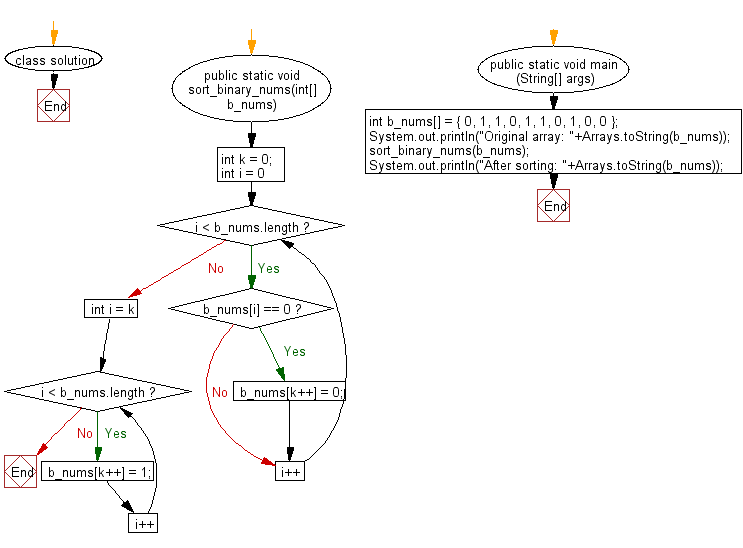﻿ Java exercises: Sort a given binary array in linear times - w3resource# Java Array Exercises: Sort a given binary array in linear times

## Java Array: Exercise-56 with Solution

Write a Java program to sort a given binary array in linear times.

From Wikipedia,
Linear time: An algorithm is said to take linear time, or O(n) time, if its time complexity is O(n). Informally, this means that the running time increases at most linearly with the size of the input. More precisely, this means that there is a constant c such that the running time is at most cn for every input of size n. For example, a procedure that adds up all elements of a list requires time proportional to the length of the list, if the adding time is constant, or, at least, bounded by a constant.
Linear time is the best possible time complexity in situations where the algorithm has to sequentially read its entire input. Therefore, much research has been invested into discovering algorithms exhibiting linear time or, at least, nearly linear time. This research includes both software and hardware methods. There are several hardware technologies which exploit parallelism to provide this. An example is content-addressable memory. This concept of linear time is used in string matching algorithms such as the Boyer–Moore algorithm and Ukkonen's algorithm.

Example:
Input :
b_nums[] = { 0, 1, 1, 0, 1, 1, 0, 1, 0, 0 }
Output:
After sorting: [0, 0, 0, 0, 0, 1, 1, 1, 1, 1]

Sample Solution:

Java Code:

``````import java.util.Arrays;

class solution
{

public static void sort_binary_nums(int[] b_nums)
{
int k = 0;

for (int i = 0; i < b_nums.length; i++)
{
if (b_nums[i] == 0) {
b_nums[k++] = 0;
}
}

for (int i = k; i < b_nums.length; i++) {
b_nums[k++] = 1;
}
}

public static void main (String[] args)
{
int b_nums[] = { 0, 1, 1, 0, 1, 1, 0, 1, 0, 0 };
System.out.println("Original array: "+Arrays.toString(b_nums));
sort_binary_nums(b_nums);
System.out.println("After sorting: "+Arrays.toString(b_nums));

}
}
```
```

Sample Output:

```
Original array: [0, 1, 1, 0, 1, 1, 0, 1, 0, 0]
After sorting: [0, 0, 0, 0, 0, 1, 1, 1, 1, 1]
```

Flowchart:Java Code Editor:

Improve this sample solution and post your code through Disqus

What is the difficulty level of this exercise?

Test your Programming skills with w3resource's quiz.

﻿

## Java: Tips of the Day

countOccurrences

Counts the occurrences of a value in an array.

Use Arrays.stream().filter().count() to count total number of values that equals the specified value.

```public static long countOccurrences(int[] numbers, int value) {
return Arrays.stream(numbers)
.filter(number -> number == value)
.count();
}
```

Ref: https://bit.ly/3kCAgLb